Get instant live expert help with Excel or Google Sheets“My Excelchat expert helped me in less than 20 minutes, saving me what would have been 5 hours of work!”

#### Post your problem and you’ll get expert help in seconds.

Your message must be at least 40 characters
Our professional experts are available now. Your privacy is guaranteed.

# How to multiply matrices in Excel – Excelchat

If you want to find the product of two matrices you have to multiply the elements of each row in matrix with 1 with the elements of each column in matrix 2. And while this might be a tedious work, matrix multiplication is an option that we should all explore. Knowing how to solve matrix problems can help you save a lot of time while performing more complicated mathematical operations. But even if you know what a matrix is, it is necessary to have a short review of how it looks like. Below is a simple reminder of how a matrix looks;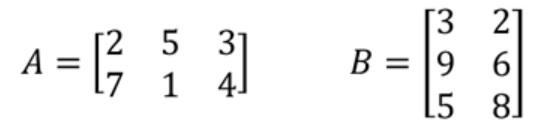Figure 1: Example of a matrix

In order to solve the above matrix, as already explained, we shall have to multiply each element in row 1 of matrix A with each element of column 1 of matrix B.

## Matrix multiplication using MMULT function

To easily multiply matrix, we can use the MMULT function. This function will return the matrix product of two arrays, like those in the figure above.

The general syntax of the formula is as shown below;

`=MMULT (array1, array2)`

Where;

• Array1- is the matrix array to multiply
• Array2- is the second matrix array to multiply.

### Example 1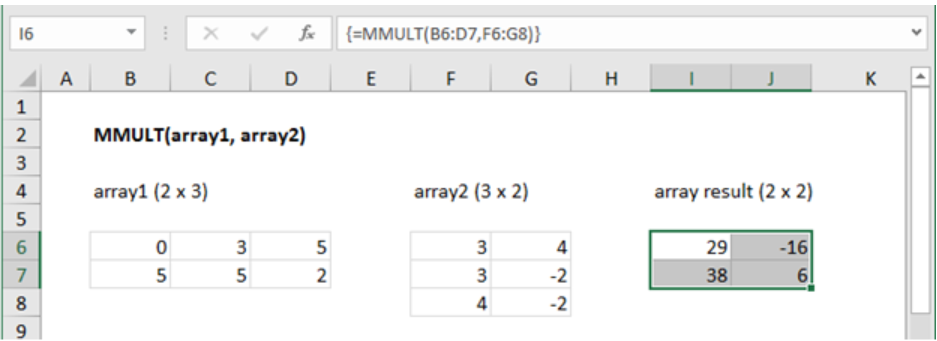Figure 2: 2 x 2 matrix multiplication

In this example, we want to multiply matrix array1 and matrix array2 using the MMULT function. To do this, enter the formula below, in an array format;

`{=MMULT (B6:D7, F6:G8)}`

To enter the above formula in an array format, you have to press Ctrl + Shift + Enter

### Example 2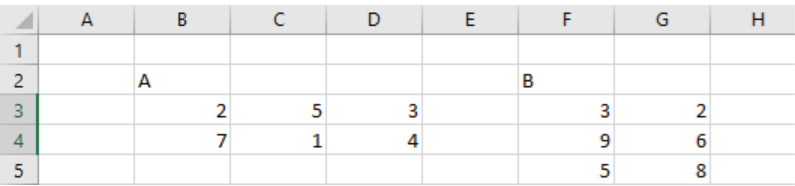Figure 3: Matrix A and B

In the above figure, we have matrix A and matrix B. and we want to find the product of the two matrices. It is important to remember that multiplying the two matrices will return a 2×2 matrix. This is because the matrix A has two rows while matrix B has 2 columns.

For us to multiply the two matrices, we first need to select an area on the worksheet where the product should be. The area should be two cells wide and two cells high.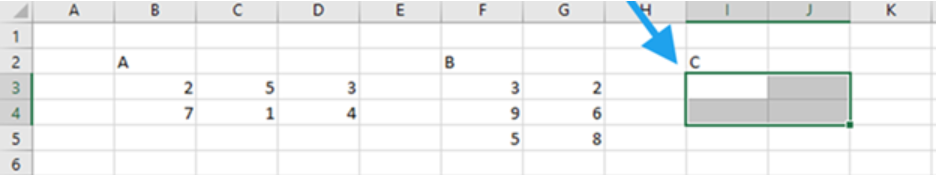Figure 4: Array result of matrix product

After selecting the area to contain the product, we now need to type in the array matrix multiplication formula.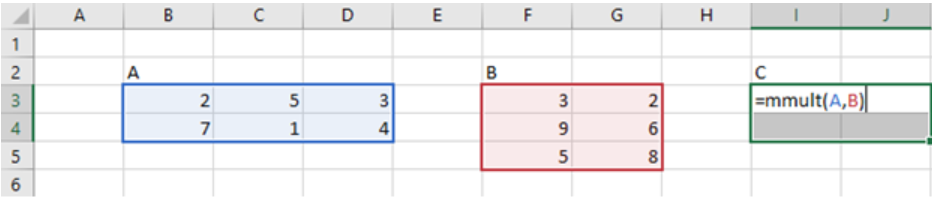Figure 5: Matrix product

The last thing we now need to do is press Ctrl + Shift + Enter to get the product of the matrices. This procedure is critical given that this is an array formula.

Once you have the matrix product it is now easy to find the determinant of the matrix as well.

## Instant Connection to an Expert through our Excelchat Service

Most of the time, the problem you will need to solve will be more complex than a simple application of a formula or function. If you want to save hours of research and frustration, try our live Excelchat service! Our Excel Experts are available 24/7 to answer any Excel question you may have. We guarantee a connection within 30 seconds and a customized solution within 20 minutes.